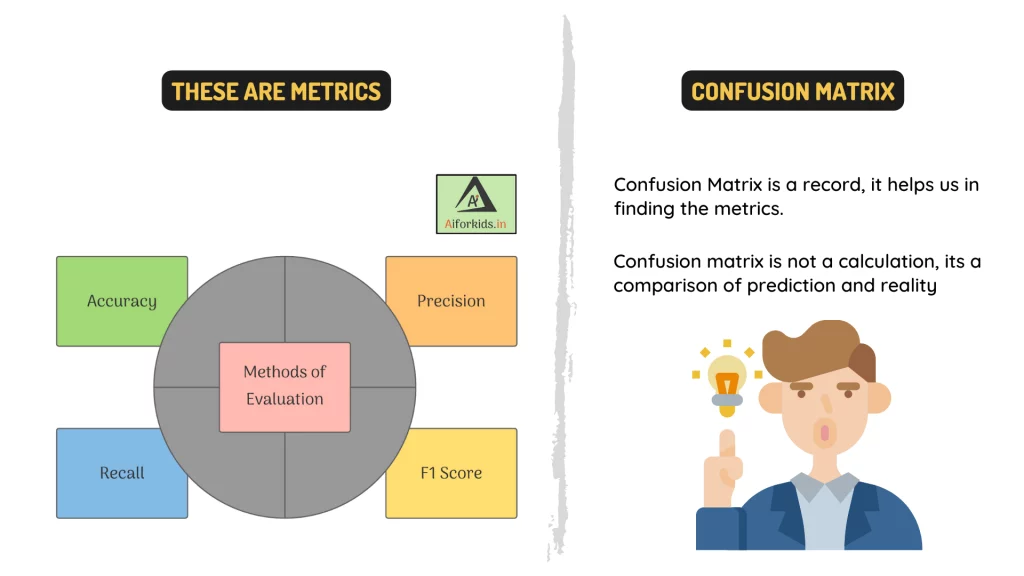# ________ is the performance measurement for machine learning classification problem where output can be 2 or more classes.

more_vert

________ is the performance measurement for machine learning classification problem where output can be 2 or more classes.

 Topic Evaluation Type Fill in the Blanks Class 10

more_vert

verified

Confusion Matrix is the performance measurement for machine learning classification problem where output can be 2 or more classes.

Explanation:There are a number of different performance measures that can be used to evaluate the performance of a classification model on a multiclass classification problem, where the output can take on two or more classes, they all together are called confusion matrix. Some common measures include:

• Accuracy: This is the most common evaluation metric for classification problems. It is the proportion of correct predictions made by the model, with respect to the total number of predictions.

• Precision: This is the proportion of true positive predictions made by the model, with respect to all positive predictions made by the model.

• Recall: This is the proportion of true positive predictions made by the model, with respect to all actual positive instances in the dataset.

• F1 Score: This is the harmonic mean of precision and recall. It is a good metric to use when you want to balance precision and recall.